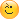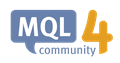# How to read angle of trend line ? - page 2

[Deleted]

It's not nonsense Raptor. It doesnt matter what the x and y axis represent as long as they are numerical

I too think its not nonsense RaptorUK.i think you may have to review your viewpoint.

....trying to work out an angle is not logical and is nonsensical.

its also true that in pure simple mathematics term,an angle is define between two lines or tangents if both are in same units.on the other hand,as one sees the chart we can easily see the steepness of a moving average/lines/trendlines and ascertain the strength/acceleration/deceleration of a trend.steeper the trend more the angle/slope.so what can be visually seen can be programmatically calculated and should have meaning! and as Einstein said Everything is relative in ..Theory of Relativity and therefore our reality is also relative and is define by mostly what we see..

I was aware of the possibility for angles in chart to be calculated and have a meaning but not exactly aware of how to set the units along the two lines required for calculating it ...this scaling factor seems to be the answer..

reality example:D

say an astronomer through a telescope sees three distant stars forming a triangle with DEFINITE ANGLES between the sides but for an observer seeing it with naked eye,the three stars is seen as just one point...problem of zooming out too far for the observer!

SDC:

It's not nonsense Raptor. It doesnt matter what the x and y axis represent as long as they are numerical.

Sorry, that is simply incorrect. Take a 3,4,5 triangle, work out the angle between the 4 & 5, it's approx 37 deg. Now take the same triangle in meters and convert the 4 to feet = 13.1' now the angle between the 13.1' and 5m is not valid, but the lengths are still numeric so what is the problem ? cos-1 4/5 works, cos-1 13.1/5 doesn't. A gradient works in both cases as the units are specified, m/m in the first case, m/feet in the second case. With an angle the units of the dimensions that make the angle are not specified so they are assumed to be the same unit . . . how will you specify an angle of 30 deg on a chart ?

You didnt read the rest of my post, you have to define which scale is the "real" one and work your calculations to that scale. Just because a book somewhere doesnt say 1 pip = 1 bar, does not mean you cant say 1pip = 1 bar. it is just a user defined corrolation.

Look at a thermometer it has two scales one says water boils at 100 another says it boils at 212. How can that be ? They are both used defined corrolations between mercury expansion and temperatrure.

SDC:

You didnt read the rest of my post, you have to define which scale is the "real" one and work your calculations to that scale.

Of course I didbut the rest doesn't make any difference to what you wrote. Answer me this question, "how will you specify an angle of 30 deg on a chart ?" you can't simply say "30 deg" because you need more information thaan that . . . so how will you specify it such that someone else will know what you mean and be able to reproduce your measurement ?

I would tell them what scale I am using. same as if you are talking about temperature you have to tell them which scale you are using.

You could say the Gann scale is 1pip = 1bar seeing as he already kinda said that with the Gann line (although you can change the scale it works to)

You could then define your own scale call it the raptor scale 20 pips = 1 bar.

"set your chart to the Raptor scale and draw a 45 degree line."

SDC:

You could say the Gann scale is 1pip = 1bar seeing as he already kinda said that with the Gann line (although you can change the scale it works to)

You could then define your own scale call it the raptor scale 20 pips = 1 bar.

"set your chart to the Raptor scale and draw a 45 degree line."

OK, yes I see how that is far simpler and less prone to error than simply stating a gradient of x points/bar or y points/timeWithin an EA or an indicator it wouldnt matter which scale you use, as long as you define the scale first and work to that scale all the subsequent calculations would be valid within that EA, so you work to the raptor scale, a 45 degree line has a real value it bisects co-ordinates (from a zero level) 1,20 2,40, 3,60, you can then say if price crosses that line and all the other things you might do in an EA.

I wasnt taling in terms of simplicity I was simply addressing the question of possibility.

Hi all, 6 years late, but I hope this solution will help you.

You can try using ChartTimePriceToXY() function.

https://docs.mql4.com/chart_operations/charttimepricetoxy

Keep in mind, the X-axis and Y-axis origin is based on TOP LEFT

Get X1-Y1 coordinate with input Time1-Price1

Get X1-Y2 coordinate with input Time2-Price2

With that, you can use mathArctan() to deduce the angle, via Right Angle Triangle analysis

https://docs.mql4.com/math/matharctan

Just be careful with the X-Y values  and your theta Positive and Negative value.

Do additional wrap around handling if required.ChartTimePriceToXY - Chart Operations - MQL4 Reference
• docs.mql4.com
ChartTimePriceToXY - Chart Operations - MQL4 Reference

MirTD:It's not nonsense Raptor. It doesnt matter what the x and y axis represent as long as they are numerical
Wrong. Can't be done.
1. There is no angle from 2 anchor points. An angle requires distance divided by distance; a unit-less number. A chart has price and time. What is the angle of going 30 miles in 45 minutes? Meaningless!

2. You can get the slope from a trendline: m=ΔPrice÷ΔTime. Changing the axes scales changes the apparent angle, the slope is constant. Angle is meaningless.

3. You can create an angled line using one point and setting the angle (Object Properties - Objects Constants - Standard Constants, Enumerations and Structures - MQL4 Reference.) The line is drawn that angle in pixels. Changing the axes scales does NOT change the angle (and thus is meaningless.)

4. If you insist, take the two price/time coordinates, convert them to pixel coordinates and then to apparent angle with arctan.
How to get the angle of a trendline and place a text object to it? - Trend Indicators - MQL4 programming forum - Page 2